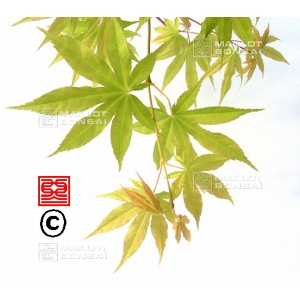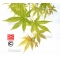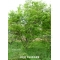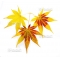##### The Japanese Bonsai specialist
Direct order Contact Help / Services Newsletter# Acer palmatum seeds jiro shidare

› Acer seeds and moreref. : 9398

6,00

Available quantity : 36Order

###### Description

Leaves: tender yellowish green with brown tips, turning to green in summer and orange in autumn. Contrary to its name 'shidare', which means 'weeping' in Japanese, this variety grows straight, with long shoots that grow out beyond the foliage.

#seeds 5.5 #acer 4.4 #shidare 3.7 #palmatum 2.8 #green 2.5 #with 2.4 #more 2.4 #jiro 2.4 #yellowish 1.9 #japanese 1.8

Formule
(( ROUND((CHAR_LENGTH(b.article_nom)-CHAR_LENGTH(REPLACE(b.article_nom, 'shidare', '')))/LENGTH('shidare')) + ROUND((CHAR_LENGTH(b.article_description)-CHAR_LENGTH(REPLACE(b.article_description, 'shidare', '')))/LENGTH('shidare')) ) * 3.7) + (( ROUND((CHAR_LENGTH(b.article_nom)-CHAR_LENGTH(REPLACE(b.article_nom, 'seeds', '')))/LENGTH('seeds')) + ROUND((CHAR_LENGTH(b.article_description)-CHAR_LENGTH(REPLACE(b.article_description, 'seeds', '')))/LENGTH('seeds')) ) * 3.5) + (( ROUND((CHAR_LENGTH(b.article_nom)-CHAR_LENGTH(REPLACE(b.article_nom, 'palmatum', '')))/LENGTH('palmatum')) + ROUND((CHAR_LENGTH(b.article_description)-CHAR_LENGTH(REPLACE(b.article_description, 'palmatum', '')))/LENGTH('palmatum')) ) * 2.8) + (( ROUND((CHAR_LENGTH(b.article_nom)-CHAR_LENGTH(REPLACE(b.article_nom, 'green', '')))/LENGTH('green')) + ROUND((CHAR_LENGTH(b.article_description)-CHAR_LENGTH(REPLACE(b.article_description, 'green', '')))/LENGTH('green')) ) * 2.5) + (( ROUND((CHAR_LENGTH(b.article_nom)-CHAR_LENGTH(REPLACE(b.article_nom, 'with', '')))/LENGTH('with')) + ROUND((CHAR_LENGTH(b.article_description)-CHAR_LENGTH(REPLACE(b.article_description, 'with', '')))/LENGTH('with')) ) * 2.4) + (( ROUND((CHAR_LENGTH(b.article_nom)-CHAR_LENGTH(REPLACE(b.article_nom, 'acer', '')))/LENGTH('acer')) + ROUND((CHAR_LENGTH(b.article_description)-CHAR_LENGTH(REPLACE(b.article_description, 'acer', '')))/LENGTH('acer')) ) * 2.4) + (( ROUND((CHAR_LENGTH(b.article_nom)-CHAR_LENGTH(REPLACE(b.article_nom, 'jiro', '')))/LENGTH('jiro')) + ROUND((CHAR_LENGTH(b.article_description)-CHAR_LENGTH(REPLACE(b.article_description, 'jiro', '')))/LENGTH('jiro')) ) * 2.4) + (( ROUND((CHAR_LENGTH(b.article_nom)-CHAR_LENGTH(REPLACE(b.article_nom, 'yellowish', '')))/LENGTH('yellowish')) + ROUND((CHAR_LENGTH(b.article_description)-CHAR_LENGTH(REPLACE(b.article_description, 'yellowish', '')))/LENGTH('yellowish')) ) * 1.9) + (( ROUND((CHAR_LENGTH(b.article_nom)-CHAR_LENGTH(REPLACE(b.article_nom, 'japanese', '')))/LENGTH('japanese')) + ROUND((CHAR_LENGTH(b.article_description)-CHAR_LENGTH(REPLACE(b.article_description, 'japanese', '')))/LENGTH('japanese')) ) * 1.8) + (( ROUND((CHAR_LENGTH(b.article_nom)-CHAR_LENGTH(REPLACE(b.article_nom, 'contrary', '')))/LENGTH('contrary')) + ROUND((CHAR_LENGTH(b.article_description)-CHAR_LENGTH(REPLACE(b.article_description, 'contrary', '')))/LENGTH('contrary')) ) * 1.8)

## Secure payment## Delivery

Our logistic partners :